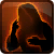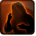Conditions

## Related effects, buffs and debuffs

• [hidden] [does not expire]Effect #1

 Slot: EffectOther Duration: passive Tick rate: does not tick # occurrences: 1
• After an ability is activated by NONE
• After an ability is activated by NONE
• After an ability is activated by NONE
• After an ability is activated by NONE

Perform the following actions:

• Modify Cooldown
- Is Percentage = (bool) true
- Tag = (string)
- Tags = (string)
- Ability Spec = (int) 16141163209394767649
- Amount Min = (float) 0
- Amount = (float) -1
• Modify Cooldown
- Is Percentage = (bool) true
- Tag = (string)
- Tags = (string)
- Ability Spec = (int) 16141056792234952351
- Amount Min = (float) 0
- Amount = (float) -1
• Unknown (193)
- Unknown (601) = (bool) false
- Unknown (602) = (bool) false
- Unknown (607) = (bool) false
- Tags = (string)
- Ability Spec = (int) 16141163209394767649
- Amount Max = (float) 1
- Amount Min = (float) 1
- Amount Percent = (float) 0
• Unknown (193)
- Unknown (601) = (bool) false
- Unknown (602) = (bool) false
- Unknown (607) = (bool) false
- Tags = (string)
- Ability Spec = (int) 16141056792234952351
- Amount Max = (float) 1
- Amount Min = (float) 1
- Amount Percent = (float) 0